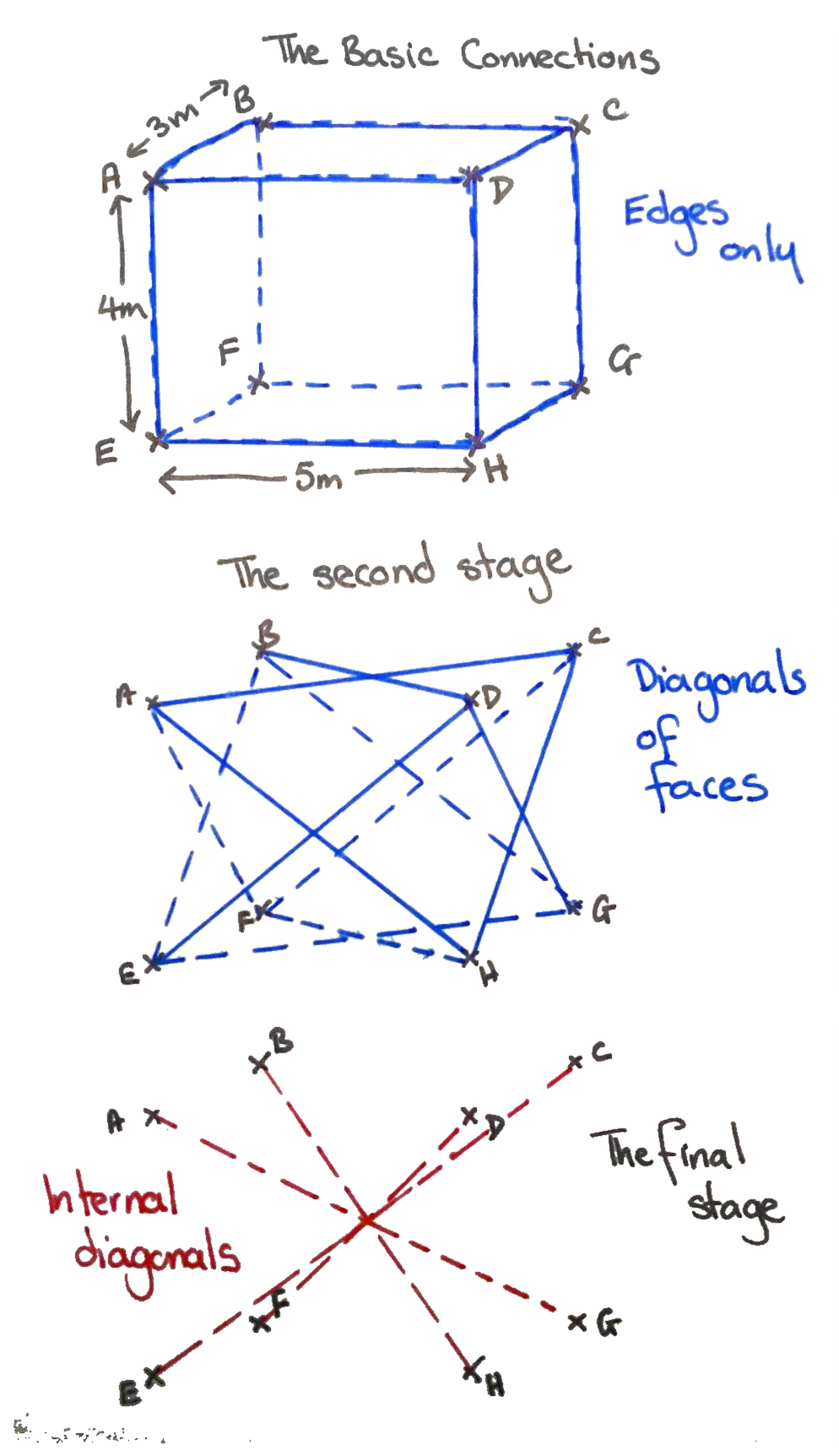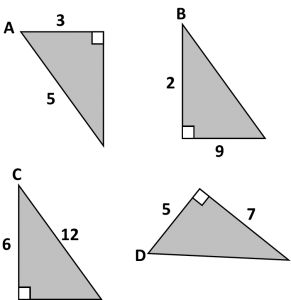# 268. Monkeying with Pythagoras

My (not so little) monkeys in KS3 have been discovering and using Pythagoras’ Theorem. They usually deal with open questions quite well, however this one took a fair bit of discussion. This challenge requires no worksheets or fancy resources, just write it on the board. The context is modified, but the essential question remains the same.

Challenge

Zookeepers have attached eight bolts in a cuboid formation (sides 3m, 4m and 5m) to the trees in a chimpanzee enclosure. The keepers attach taut ropes between the bolts for the chimps to climb on. Each length of rope is individually cut. No length is lost in knots.

• What is the maximum length of any one piece of rope?
• What is the total amount used, if every corner is joined without duplication?

Solution

The first step to solving it is a good diagram of the problem. Students then need to break it down into triangles. The solution has several levels of difficulty:

• Total of the edges of the cuboid
• Total of the diagonals on the faces
• Total of the diagonals across the inside of the cuboid

This diagram demonstrates the levels of the problem – have fun!# 237. Quick Starter

Don’t you just hate it when students forget basic key skills? Especially those at the higher end of Year 11 or studying A-Level, who should have a better core knowledge. What if there was a magic tool which began to address this issue?

Skills required

• Comparing fractions
• Trigonometric ratios
• Simplifying surds
• Rationalising surds
• Pythagoras

Equipment

You will not need:

• Worksheet
• Powerpoint
• Printer
• Laminator
• Calculator

Magic Tool

• One board, with pen

Activity

Quite simply draw the four diagrams below on the board and ask the following questions:1. Which has the largest sine ratio: A or B?
2. Which has the largest cosine ratio: C or D?
3. Which has the smallest tangent ratio: A, B, C or D?
4. Extension: Calculate the missing angles and areas (Calculator allowed)

It takes moments to draw the questions on the board, but the discussion can take some time and addresses several basic skills. You can change the numbers to adjust the level of challenge.